• 语言为C++，使用了set，map容器，输入格式：S -> Aa | g | e，支持多‘|’ 符号，采用文件输入
• 输入任意的上下文无关文法,输出所输入的上下文无关文法一切非终结符的first集合和follow集合 输入任意的上下文无关文法,输出所输入的上下文无关文法一切非终结符的first集合和follow集合
• C语音代码。实现功能：1....2.求每个非终结符FIRST 集FOLLOW集和SELECT集模块。3.预测分析表的构建模块。4.文法的检验及消除左公因子和左递归模块。5.对输入终结符串的判断，是否为LL1文法，并进一步分析。select
• 这是鄙人完成老师的一个实验作业，写的还不错已比较详细，且代码中有大量注释帮助理解。使用时打开vc，在文件里选择打开工作区间，打开文件里的test2.dsw即可使用。里面的大量过程结果被鄙人注释掉了，打开1.cpp里的...
• 编译原理实验报告实验内容： 1.求出每个非终结符的FIRST集合 2.求出每个产生式右部的FIRST集合 3.求出每个非终结符的Follow集合 实验环境： Visual Studio2010 实验目的: 让同学们掌握FIRST集合和FOLLOW集合的求法...WORD
• 编译原理上机实验系列（2） 一、预习准备 1.实验目的 掌握First和Follow集合求法，深入理解LL(1)文法分析过程。 2.实验要求 根据文法求出First和Follow集合 3.实验环境 Xcode 二、实验过程 1.实验步骤 （1）First集 ...


文章目录
前言一、预习准备1.实验目的2.实验要求3.实验环境
二、实验过程1.实验步骤（1）First集（2）Follow集
2.实验数据记录3.实验代码
三、实验总结1.数据处理2.实验结果3.心得体会
参考文献

前言
编译原理上机实验系列（2）
一、预习准备
1.实验目的
掌握First和Follow集合求法，深入理解LL(1)文法分析过程。
2.实验要求
根据文法求出First和Follow集合
3.实验环境
Xcode
二、实验过程
1.实验步骤
（1）First集
假设 α–>tβ，求FIRST(α): ①如果首符号t是终结符则直接放入first集合中 ②如果t不是非终结符： 如果t—>ε，则将ε加入FIRST(α)，并且将β进行①②操作判断。 如果t不是ε，那么求first(t)，并将first(t)的结果放入FIRST(α)中。
（2）Follow集
假设A -->αBβ，A -->αB，求follow（B）: ①如果A是开始符, 将加入$FOLLOW(A), 这里$是输入结束标记. ②对于A -->αBβ，首先判断β是否可以推出ε 如果不能推出ε，那么将first（β）加入follow（B） 如果推出ε，那么将{ first(β)- ε } U follow(A)加入follow（B） ③对于A -->αB，将follow(A)结果加入follow（B）。
2.实验数据记录
待输入数据：
4
S->ABC
A->a
B->@
C->c

预期结果：
first[S] = a
first[A] = a
first[B] = @
first[C] = c
follow[S] = #
follow[A] = c
follow[B] = c
follow[C] = #

3.实验代码
在文法G[S]中使用’@’代表空。
//
//  main.cpp
//  exam2
//
//  Created by 小仙女 on 2021/6/7.
//

#include <cstdio>
#include <cstring>
#include <map>
#include <stack>
#include <algorithm>

using namespace std;

struct node
{
char s1, s2;
}g;

char s;
int num;
map<char, int> Map1;
map<char, int> Map2;

int Map1_num;
int Map2_num;

char s1, s2;

int first;
int follow;

int vis;
int acc;

int select1;

int c;
char sta;

int cnt;

void dfs1(char ch, int dis[])
{
if(acc[Map1[ch]])
{
for(int i = 1;i<=Map2_num;i++)
{
dis[i] = first[Map1[ch]][i];
}
return;
}
int value;
memset(value, 0, sizeof(value));
for(int i = 1;i<=num;i++)
{
if(vis[i]) continue;
if(g[i].s1!=ch) continue;
int j, k, len = strlen(g[i].s2);
for(j = 0;j<len;j++)
{
if(Map1[g[i].s2[j]])
{
vis[i] = 1;
dfs1(g[i].s2[j], value);
for(k = 2;k<=Map2_num;k++)
{
if(value[k]) dis[k] = 1;
}
if(value == 0) return;
}
else
{
dis[Map2[g[i].s2[j]]] = 1;
break;
}
}
if(j == len)
{
dis = 1;
}
}
return;
}

void dfs2(char ch, int dis[])
{
int i, j, k, len, flag = 0;
if(acc[Map1[ch]])
{
for(int i = 1;i<=Map2_num;i++)
{
dis[i] = follow[Map1[ch]][i];
}
return;
}
for(i = 1;i<=num;i++)
{
len = strlen(g[i].s2);
for(j = 0;j<len;j++)
{
if(g[i].s2[j] == ch)
{
flag = 1;
continue;
}
if(!flag) continue;
if(Map1[g[i].s2[j]])
{
for(k = 2;k<=Map2_num;k++)
{
if(first[Map1[g[i].s2[j]]][k])
{
dis[k] = 1;
}
}
if(!first[Map1[g[i].s2[j]]])
{
flag = 0;
}
}
else
{
dis[Map2[g[i].s2[j]]] = 1;
flag = 0;
}
}
if(!flag||vis[i]) continue;
int value;
memset(value, 0, sizeof(value));
vis[i] = 1;
dfs2(g[i].s1, value);
for(i = 1;i<=Map2_num;i++)
{
if(value[i]) dis[i] = 1;
}
}
}

int main()
{
int i, j, len;

num = Map1_num = Map2_num = 0;
Map1.clear();
Map2.clear();

Map2['@'] = ++Map2_num;
s2[Map2_num] = '@';
int m;
scanf("%d", &m);
while(m--)
{
scanf("%s", s);
len = strlen(s);
num++;
for(i = 0;i<1;i++)
{
g[num].s1[i] = s[i];
if(!Map1[s[i]])
{
Map1[s[i]] = ++Map1_num;
s1[Map1_num] = s[i];
}
}

g[num].s1[i] = '\0';
for(i = 3;i<len;i++)
{
g[num].s2[i-3] = s[i];
if(s[i]>='A'&&s[i]<='Z')
{
if(!Map1[s[i]])
{
Map1[s[i]] = ++Map1_num;
s1[Map1_num] = s[i];
}
}
else
{
if(!Map2[s[i]])
{
Map2[s[i]] = ++Map2_num;
s2[Map2_num] = s[i];
}
}
}
g[num].s2[i-3] = '\0';
}

memset(first, 0, sizeof(first));
memset(acc, 0, sizeof(acc));

for(i = 1;i<=Map1_num;i++)
{
int value;
memset(vis,0,sizeof(vis));
memset(value,0,sizeof(value));
dfs1(s1[i],value);
for(j = 1 ; j <= Map2_num; j++)
{
first[i][j] = value[j] ;
}
acc[i] = 1 ;
printf("first[%c] = ", s1[i]);
for(j = 1 ; j <= Map2_num ; j++)
{
if( first[i][j] )
{
printf("%c ", s2[j]);
}
}
printf("\n");
}

Map2['#'] = ++Map2_num ;
s2[ Map2_num ] = '#' ;
memset(follow,0,sizeof(follow));
memset(acc,0,sizeof(acc));
for(i = 1 ; i <= Map1_num ; i++)
{
int value ;
memset(vis,0,sizeof(vis)) ;
memset(value,0,sizeof(value));
if( s1[i] == 'S' )
{
value[ Map2['#'] ] = 1 ;
}
dfs2(s1[i],value);
for(j = 1 ; j <= Map2_num; j++)
{
follow[i][j] = value[j] ;
}
acc[i] = 1 ;
printf("follow[%c] = ", s1[i]);
for(j = 1 ; j <= Map2_num ; j++)
{
if( follow[i][j] )
{
printf("%c ", s2[j]);
}
}
printf("\n");
}
return 0;
}


三、实验总结
1.数据处理
Follow一般从上往下找，而且第一轮的时候有时候不能够将所有的follow都填满，所以要采用迭代的方式不断循环。 有时候遇到闭环的时候，先假设按照第一遍把所有的都算出来后，第二轮的时候将所有集合制空，如果有变化，则在进行一轮，直到没有变化为止。
2.实验结果
console输出结果如下：3.心得体会
本次实验我通过实践学习了LL(1)文法中first集合follow集合的构造方法、掌握了相关的算法，对课堂上的知识学以致用，同时通过调试对汇编编译过程也有了一定了解，程序运行结果正确符合题意，总体来说受益匪浅。
参考文献
https://blog.csdn.net/shadowam/article/details/84193278 
展开全文c++
• 通过设计、开发一个高级语言的LL（1）语法分析程序，实现 对源程序的语法检查和结构分析，括自顶向下语法分析、First集、Follow集、Select集、文法等价变换）的理解，提高语法分析方法的实践能力。
• 编译原理实验 first、follow、select集合的求解，经测试正确，c语言编写follow
• 编译原理之NULL集、first、follow集C语言实现，实现中句子的转换符号由‘#’代替，数组默认由‘*’作为结束符
• 首先这是我 看了一下午 搜了好多视频好不容易总结的（也是好不容易看懂的o(╥﹏╥)o） 文章目录 首先这是我 看了一下午 搜了好多视频好不容易总结的（也是好不容易看懂的o(╥﹏╥)o） 1、写在前面： 2、*FIRST集 我...
首先这是我 看了一下午 搜了好多视频好不容易总结的（也是好不容易看懂的o(╥﹏╥)o）

文章目录
首先这是我 看了一下午 搜了好多视频好不容易总结的（也是好不容易看懂的o(╥﹏╥)o）1、写在前面：

2、*FIRST集我觉得直接上题开始讲解才是很容易理解的反正这是我自己的总结 防止我以后忘接了 嘻嘻(# ^ . ^#)在给定的文法中按照顺数来依次找 ==找给定产生式的第一个终结符依次向下找，因为找的是首符集（first）==

这就是我总结的first集的写法
3、FOLLOW集算法没错所有的精华就在这几句话中
4、为什么要引入follow集5. 这是这道题的SELECT集
看到最后的帮忙点个==赞==👍🙏 谢谢！

![在这里插入图片描述](https://img-blog.csdnimg.cn/20200129163341581.png )

1、写在前面：
首先要知道什么是终结符，什么是非终结符
我理解的就是 可以再向下分解的就是非终结符（例如：T B A…之类的） 反之不可以继续分解的就是终结符（例如：a b c ‘（‘ ’） ’ [ ’ ’ ] ’ ）也可以说 大写字母是非终结符 可以继续分解的 而 终结符是 小写字母 或者是 一些符号 不可以分解的

例题：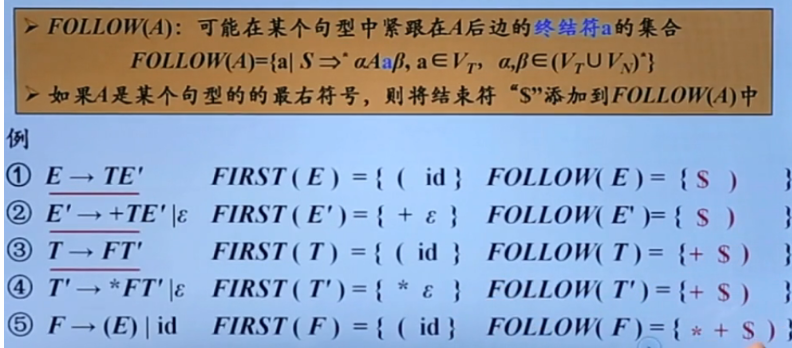2、*FIRST集
我觉得直接上题开始讲解才是很容易理解的
反正这是我自己的总结 防止我以后忘接了 嘻嘻(# ^ . ^#)

. 首先根据给定的文法 1. E —> T E ’ 2. E ’ —> + T E ’ | ε 3. T —> F T ’ 4. T ’ —> * F T ’ | ε 5. F —> (E) | id
首先从E开始 开始都有一个# 或 $(我也不知道是哪个 看到好几个版本蒙了 我就用$ 吧 代表结束符)
（读者首先应注意到用作标记输入结束的“ #”，它就像是 Foll ow 集合计算中的一个记号。若没有它，那么在整个被匹配的串之后就没有符号了。由于这样的串是由文法的开始符号生成的，所以#必须总是要增加到开始符号的 Follow 集合中。）
在给定的文法中按照顺数来依次找 找给定产生式的第一个终结符依次向下找，因为找的是首符集（first）
FIRST（E）= { $} 1.E —> T E ’ ----------> 3. T —> F T '----->5. F —> ( E ) | id 所以FIRST（E）= { （，id } 2.E ’ —> + T E ’ | ε—因为开始就是终结符 所以FIRST（E '）= { + , ε } 3.T —> F T '------>5. F —> ( E ) | id 所以FIRST（ T ）= { （，id } 4.T ’ —> * F T ’ | ε------因为开始就是终结符 所以FIRST（ T ’ ）= { * ，ε } 6. F —> ( E ) | id-----因为开始就是终结符 所以FIRST（ F ）= { （ ，id } 这就是我总结的first集的写法 3、FOLLOW集 follow集呢其实有一个算法 刚开始你可能看不懂 不是道什么意思 但是当你真正明白的时候 你会发现他说的都是对的-----难顶呀！ 算法 集合 Follow (A) 的定义如下： （1）若 A 是开始符号，则#就在 Follow (A) 中。 （2）若存在产生式B →aAg ，则First (g) - {ε }在 Follow (A) 中。 （3）若存在产生式B →aAg ，且ε在 First (g) 中，则 Follow (A)包括 Follow (B)。 没错所有的精华就在这几句话中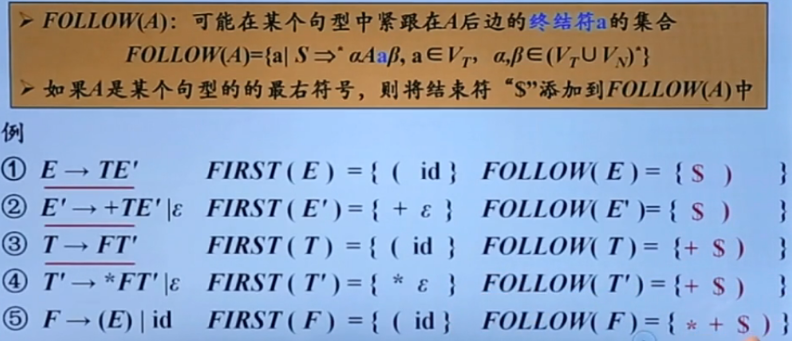(这是刚才的题 我再拿过来 上下翻麻烦) 一定要耐心的看这几句话 我写的很认真的 你认真看一定能明白的！加油ヾ(◍°∇°◍)ﾉﾞ。 1. 首先看开始符E 默认开始符E的 follow集中存在$(#)结束符 然后在产生式的右部分找有没有E了，然后看见5中有E ，E后面的是终结符 ')' 所以就加在FOLLOW（E）={ $, ) }中 2. 再看E' ，在文法的右部分找有E'的产生式 找到1和2 因为E'是句型的最右符号 所以follow(E') 包括follow(E) 所以follow(E') ={$ , ) } 和E一样
3. 再看T，在文法的右部分找有T的产生式 找到1和2，因为T后面是E',又因为E' 的first集是{+，ε } ，所以可以是+或ε首先+可以 放在follow（T）集中，然后又因为E'可能是ε 空产生式，所以follow(T)包括follow(E)所以将{ $, ) }放在follow(T)集中所以follow(T)={+，$ , ) }
4. 再看T' , 在文法的右部分找有T'的产生式,找到3和4，因为T'是句型的最右符号，所以follow(T') 包括follow(T)所以follow(T') ={ +， $, ) } 和T一样 5. 最后看F，在文法的右部分找有F的产生式，找到3和4，因为F后面是T',而T'的首符集（follow集）是{*，ε }所以 ‘ * ’放在follow(F)集中，然后T'还有可能是空产生式ε，所以follow(F)包括follow(T)所以将{+，$ , ) }放在follow(T)集中，所以最后follow(F) ={ *，+， $, ) } 啊啊啊好累啊 一个一个字码的！ 自己写的自己应该能看懂 嘻嘻 4、为什么要引入follow集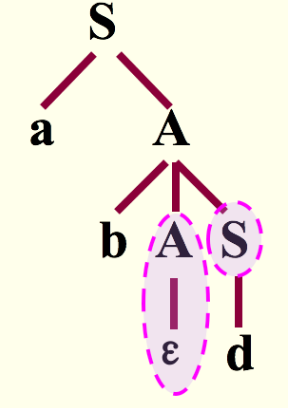这一句帮助理解为什么要引入follow集 要知道前因后果 因为在自顶向下的分析过程中 如果当某一非终结符（A）的产生式中含有空产生式ε时（如：A—>ε）则找不到继续向下的式子了，但是呢之后的S式子中有我们要找的终结符d 由此我们引入了follow集，用来找寻因为空产生式ε所造成的问题。因此，引入了一个文法符号的后跟符号集合follow集。 5. 这是这道题的SELECT集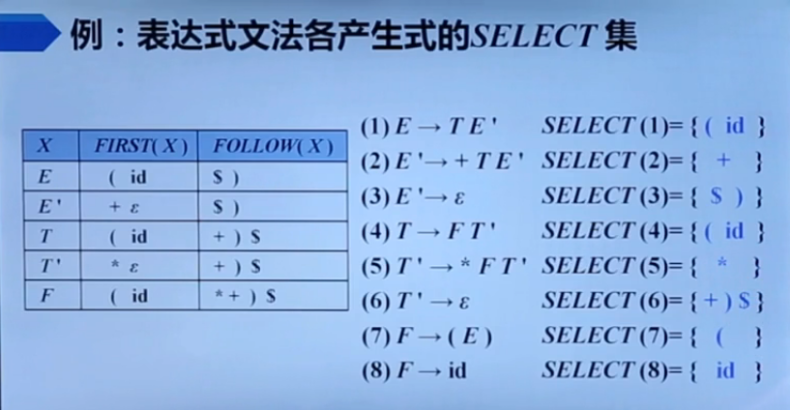first集和follow集理解了 select集看图就很容易理解了 将文法的合起来的产生式都分开变成单独的一条没有遇到ε空产生式的就用对应的first集遇到ε空产生式就用对应的follow集ok 完美 看到最后的帮忙点个赞👍🙏 谢谢！展开全文java • 文章目录FIRST集的求法FOLLOW集的计算生成预期分析表算法例题 FIRST集的求法 FIRST集是一个文法符号串所可能推导出的符号串的第一个终结符的集合 对于文法G的任一符号串α\alphaα = x1x2…xnx_{1}x_{2} \ldots x_{n...  文章目录 FIRST集的求法FOLLOW集的计算生成预期分析表算法例题分析句子 FIRST集的求法 FIRST集是一个文法符号串所可能推导出的符号串的第一个终结符的集合 对于文法G的任一符号串 α \alpha = x 1 x 2 … x n x_{1}x_{2} \ldots x_{n} 置 FIRST( α \alpha ) = ϕ \phi 将 FIRST( x 1 x_{1} ) 中一切非 ϵ \epsilon 符号加进 FIRST( α \alpha )若 ϵ ∈ \epsilon\in FIRST( x 1 x_{1} ), 将 FIRST( x 2 x_{2} ) 中一切非 ϵ \epsilon 符号加进FIRST( α \alpha ); 若 ϵ ∈ \epsilon \in FIRST( x 1 x_{1} ) 和 FIRST( x 2 x_{2} ), 将 FIRST( x 3 x_{3} ) 中一切非 ϵ \epsilon 符号加进 FIRST( α \alpha ) 中, 依此类推.对于一切 1 ⩽ \leqslant i ⩽ \leqslant n, ϵ ∈ \epsilon \in FIRST( x i x_{i} ), 则将 ϵ \epsilon 符号加入 FIRST( α \alpha ). note: FIRST集从产生式左侧推导，例如求A的FIRST集，要先从产生式左侧找到A，然后根据产生式右侧的信息求出A的FIRST集 例如： S → \rightarrow BS ‘ ^ S ‘ → ^ \rightarrow aB | ϵ \epsilon B → \rightarrow DB ‘ ^ B ‘ → ^ \rightarrow b | ϵ \epsilon D → \rightarrow d | ϵ \epsilon FIRST(S) = {d, b, a, ϵ \epsilon } FIRST(S ‘ ^ ) = {a, ϵ \epsilon } FIRST(B) = {d, b, ϵ \epsilon } FIRST(B ‘ ^ ) = {b, ϵ \epsilon } FIRST(D) = {d, ϵ \epsilon } 解释： 第一个求S的FIRST集，先从产生式的左侧找到S, (S → \rightarrow BS ‘ ^ ), 然后根据该产生式右侧的信息(BS ‘ ^ ) 和求FIRST集的第二条规则求FIRST(B), 从产生式的左侧找到B, (B → \rightarrow DB ‘ ^ ), 然后根据该产生式右侧的信息(DB ‘ ^ ) 和求FIRST集的第二条规则求FIRST(D), 从产生式的左侧找到D, (D → \rightarrow d | ϵ \epsilon ), 然后根据该产生式右侧的信息(d | ϵ \epsilon ) 和求FIRST集的第二条规则, 将终结符d加入FIRST(S). F I R S T ( S ) = { d } \color{red} { FIRST(S) = \{d\} } 又根据第三条规则，因为D → ϵ \rightarrow \epsilon , 所以将FIRST(B ‘ ^ ) 加入到FIRST(S)中，从产生式的左侧找到B ‘ ^ , (B ‘ → ^ \rightarrow b | ϵ \epsilon ), 然后根据该产生式右侧的信息(b | ϵ \epsilon ) 和求FIRST集的第二条规则, 将终结符b加入FIRST(S). F I R S T ( S ) = { d , b } \color{red} { FIRST(S) = \{d, b\} } 又根据第三条规则，因为B ‘ → ϵ ^ \rightarrow \epsilon , 所以将FIRST(S ‘ ^ ) 加入到FIRST(S)中，从产生式的左侧找到S ‘ ^ , (S ‘ → ^ \rightarrow aB | ϵ \epsilon ), 然后根据该产生式右侧的信息(aB | ϵ \epsilon ) 和求FIRST集的第二条规则, 将终结符a加入FIRST(S). F I R S T ( S ) = { d , b , a } \color{red} { FIRST(S) = \{d, b, a\} } 又根据第四条规则，因为 ϵ ∈ \epsilon \in FIRST(D) , ϵ ∈ \epsilon \in FIRST(B ‘ ^ ), 所以 ϵ ∈ \epsilon \in FIRST(B), 又因为 ϵ ∈ \epsilon \in FIRST(S ‘ ^ ), 所以 ϵ ∈ \epsilon \in FIRST(S). 最终结果如下: F I R S T ( S ) = { d , b , a , ϵ } \color{red} { FIRST(S) = \{d, b, a, \epsilon\} } 第二个求S ‘ ^ 的FIRST集, 先从产生式左侧找到S ‘ ^ , (S ‘ → ^ \rightarrow aB | ϵ \epsilon ), 然后根据产生式右侧的信息(aB | ϵ \epsilon ), S既能推导出aB又能推导出 ϵ \epsilon , 所以根据规则二和规则四得出: F I R S T ( S ‘ ) = { a , ϵ } \color{red}{ FIRST(S^) = \{a, \epsilon\} } 第三个求B的FIRST集, 产生式的左侧找到B, (B → \rightarrow DB ‘ ^ ), 然后根据该产生式右侧的信息(DB ‘ ^ ) 和求FIRST集的第二条规则求FIRST(D), 从产生式的左侧找到D, (D → \rightarrow d | ϵ \epsilon ), 然后根据该产生式右侧的信息(d | ϵ \epsilon ) 和求FIRST集的第二条规则, 将终结符d加入FIRST(B). F I R S T ( B ) = { d } \color{red} { FIRST(B) = \{d\} } 又根据第三条规则，因为D → ϵ \rightarrow \epsilon , 所以将FIRST(B ‘ ^ ) 加入到FIRST(B)中，从产生式的左侧找到B ‘ ^ , (B ‘ → ^ \rightarrow b | ϵ \epsilon ), 然后根据该产生式右侧的信息(b | ϵ \epsilon ) 和求FIRST集的第二条规则, 将终结符b加入FIRST(B). F I R S T ( B ) = { d , b } \color{red} { FIRST(B) = \{d, b\} } 又根据第四条规则，因为 ϵ ∈ \epsilon \in FIRST(D) , ϵ ∈ \epsilon \in FIRST(B ‘ ^ ), 所以 ϵ ∈ \epsilon \in FIRST(B), 最终结果如下: F I R S T ( B ) = { d , b , ϵ } \color{red} { FIRST(B) = \{d, b, \epsilon\} } 求第四个B ‘ ^ 的FIRST集和第五个求D的FIRST集同求第二个求S ‘ ^ 的FIRST集，结果为: F I R S T ( B ‘ ) = { b , ϵ } F I R S T ( D ) = { d , ϵ } \color{red} { FIRST(B^) = \{b, \epsilon\} }\\ { FIRST(D) = \{d, \epsilon\} } FOLLOW集的计算 FOLLOW集是文法符号后面可能跟随的终结符的集合(不包括空串) 对于文法的开始符号S，置 # 于 FOLLOW(S)中.若 A → \rightarrow aB β \beta 是一个产生式， 则把 FIRST( β \beta ) \ | ϵ \epsilon | 加至 FOLLOW(B) 中.若 A → \rightarrow aB 是一个产生式，或 A → \rightarrow aB β \beta 是一个产生式而 β ⇒ ϵ \beta \Rightarrow \epsilon (即 ϵ ∈ \epsilon \in ) FIRST(B), 则把 FOLLOW(A) 加至 FOLLOW(B) 中. note: FOLLOW集中没有 ϵ \epsilon FOLLOW集从产生式右侧推导，例如求A的FOLLOW集时，要从产生式右侧找到A，然后根据A右侧符号信息，求出A的FOLLOW集 例如： S → \rightarrow BS ‘ ^ S ‘ → ^ \rightarrow aB | ϵ \epsilon B → \rightarrow DB ‘ ^ B ‘ → ^ \rightarrow b | ϵ \epsilon D → \rightarrow d | ϵ \epsilon FOLLOW(S) = {#} FOLLOW(S ‘ ^ ) = {#} FOLLOW(B) = FIRST(S ‘ ^ ) \ | ϵ \epsilon | ∪ \cup FOLLOW(S ‘ ^ ) ∪ \cup FOLLOW(S) = {a, #} FOLLOW(B ‘ ^ ) = FOLLOW(B) = {a, #} FOLLOW(D) = FIRST(B ‘ ^ ) \ | ϵ \epsilon | ∪ \cup FOLLOW(B) = {b, a, #} 解释： 第一个求S的FOLLOW集, 因为S是开始符号，所以将#加入到FOLLOW(S)中，从产生式右侧找S，没有找到，所以最终结果: F O L L O W ( S ) = { # } \color{red} { FOLLOW(S) = \{ \# \} } 第二个求S ‘ ^ 的FOLLOW集, 从产生式右侧找S ‘ ^ , (S → \rightarrow BS ‘ ^ ), 根据右侧符号信息和求FOLLOW集的第三条规则，将 FOLLOW(S) 加入到 FOLLOW(S ‘ ^ ) 中，最终结果: F O L L O W ( S ‘ ) = { # } \color{red} { FOLLOW(S^) = \{ \# \} } 第三个求B的FOLLOW集，从产生式右侧找到B，(S → \rightarrow BS ‘ ^ 、S ‘ → ^ \rightarrow aB | ϵ \epsilon ), 根据 S → \rightarrow BS ‘ ^ 和第二条规则，得: F O L L O W ( B ) = F I R S T ( S ‘ ) ∖ ∣ ϵ ∣ = { a } \color{red} { FOLLOW(B) = FIRST(S^) \setminus |\epsilon| = \{a\} } 根据S ‘ → ^ \rightarrow aB 和第三条规则，得: F O L L O W ( B ) = F O L L O W ( S ‘ ) = { a , # } \color{red} { FOLLOW(B) = FOLLOW(S^) = \{a, \# \} } 又因为S ‘ → ϵ ^ \rightarrow \epsilon , 根据S → \rightarrow BS ‘ ^ 和第三条规则，得: F O L L O W ( B ) = F O L L O W ( S ) = { a , # } \color{red} { FOLLOW(B) = FOLLOW(S) = \{a, \#\} } 第四个求B ‘ ^ 的FOLLOW集，从产生式的右侧找到B ‘ ^ , (B → \rightarrow DB ‘ ^ ), 根据第三条规则，得: F O L L O W ( B ‘ ) = F O L L O W ( B ) = { a , # } \color{red} { FOLLOW(B^) = FOLLOW(B) = \{a, \#\} } 第五个求D的FOLLOW集，从产生式的右侧找到D, (B → \rightarrow DB ‘ ^ ), 根据第二条规则，得: F O L L O W ( D ) = F I R S T ( B ‘ ) ∖ ∣ ϵ ∣ = { b } \color{red} { FOLLOW(D) = FIRST(B^) \setminus |\epsilon| = \{b\} } 又因为B ‘ → ϵ ^ \rightarrow \epsilon , 根据 B → \rightarrow DB ‘ ^ 和第三条规则，得: F O L L O W ( D ) = F O L L O W ( B ) = { b , a , # } \color{red} { FOLLOW(D) = FOLLOW(B) = \{b, a, \#\} } 生成预期分析表算法 对于文法G的每个产生式 A → α \rightarrow \alpha , 执行第2步和第3步对每个终结符a ∈ \in FIRST( α \alpha ), 把 A → α \rightarrow \alpha 加至 M[A, a]中若 ϵ ∈ \epsilon \in FIRST( α \alpha ), 则对任何b ∈ \in FOLLOW(A) 把 A → α \rightarrow \alpha 加至 M[A, b]中 note: 每个产生式的意思是将所有含“|”的产生式全部展开，然后看每个产生式的FIRST集，如果这个FIRST集中有“ ϵ \epsilon ”, 那就要将这个产生式加入到 FOLLOW集中相应符号中 例题 已知文法G如下: S → \rightarrow AB A → \rightarrow Ba | ϵ \epsilon B → \rightarrow Db | D D → \rightarrow d | ϵ \epsilon (1) 构建文法G的LL(1)分析表 (2) 判断句子adb是否为有效文法产生的语言，并给出分析过程 因为 S ⇒ \Rightarrow A ⇒ \Rightarrow B ⇒ \Rightarrow D ⇒ ̸ \Rightarrow \not S, 所以不存在左递归 因为将 A → \rightarrow Ba | ϵ \epsilon 代入到 S → \rightarrow AB 会含有回溯，B → \rightarrow Db | D 这条产生式本身也含有回溯，所以要先消除回溯 消除左递归(本题不需要消除左递归)： P的产生式: P → \rightarrow Pa 1 _1 | Pa 2 _2 | … | Pa m _m | β 1 \beta_1 | β 2 \beta_2 | … | β n \beta_n 其中，每个a不等于 ϵ \epsilon , 每个 β \beta 不以P开头 P → β 1 P ‘ \rightarrow \beta_1 P^ | β 2 P ‘ \beta_2 P^ | … | β n P ‘ \beta_n P^ P ‘ → a 1 P ‘ ^ \rightarrow a_1 P^ | a 2 P ‘ a_2 P^ | … | a m P ‘ a_m P^ 消除回溯(提左因子) A的规则是: A → δ β 1 \rightarrow \delta \beta_1 | δ β 2 \delta \beta_2 | … | δ β n \delta \beta_n | γ 1 \gamma_1 | γ 2 \gamma_2 | … | γ m \gamma_m 其中，每个 γ \gamma 不以 δ \delta 开头 A → δ A ‘ \rightarrow \delta A^ | γ 1 \gamma_1 | γ 2 \gamma_2 | … | γ m \gamma_m A ‘ → β 1 ^ \rightarrow \beta_1 | β 2 \beta_2 | … | β n \beta_n 通过消除回溯，将文法改写为: S → \rightarrow BS ‘ ^ S ‘ → ^ \rightarrow aB | ϵ \epsilon B → \rightarrow DB ‘ ^ B ‘ → ^ \rightarrow b | ϵ \epsilon D → \rightarrow d | ϵ \epsilon 通过拆分上边的产生式，得到： S → \rightarrow BS ‘ ^ S ‘ → ^ \rightarrow aBS ‘ → ϵ ^ \rightarrow \epsilon B → \rightarrow DB ‘ ^ B ‘ → ^ \rightarrow bB ‘ → ϵ ^ \rightarrow \epsilon D → \rightarrow dD → ϵ \rightarrow \epsilon 先看这八个产生式的FIRST集，如果FIRST集中有 ϵ \epsilon , 就要看相应产生式的FOLLOW集(例如产生式3、6、8) 终结符: {a, b, d, #} 非终结符: {S, S ‘ ^ , B, B ‘ ^ , D} FIRST(S) = {a, b, d, ϵ \epsilon } FOLLOW(S) = {#} FIRST(S ‘ ^ ) = {a, ϵ \epsilon } FOLLOW(S ‘ ^ ) = {#} FIRST(B) = {d, b, ϵ \epsilon } FOLLOW(B) = {a, #} FIRST(B ‘ ^ ) = {b, ϵ \epsilon } FOLLOW(B ‘ ^ ) = {a, #} FIRST(D) = {d, ϵ \epsilon } FOLLOW(D) = {b, a, #}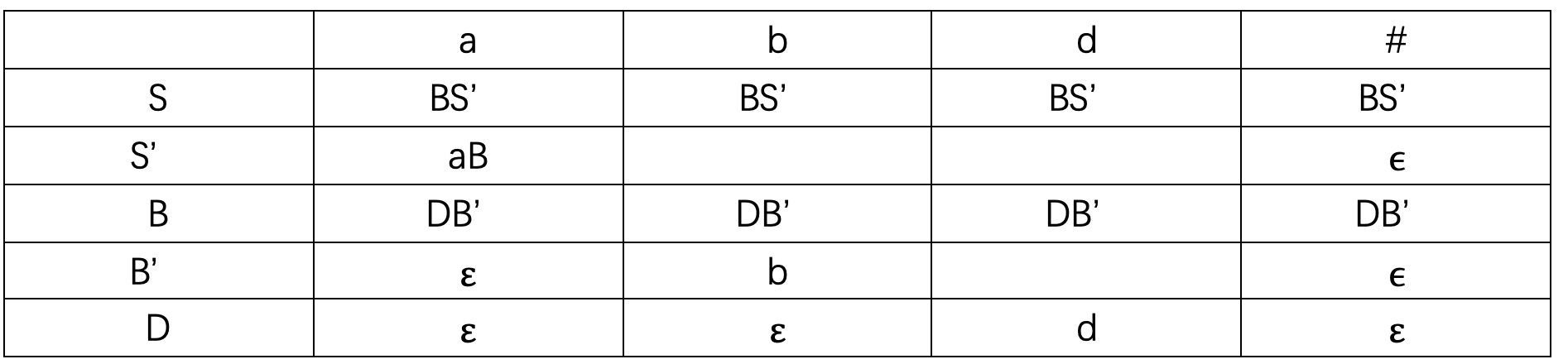解释： 先看S这一行，因为第一条产生式 FIRST(BS ‘ ^ ) = {a, b, d, ϵ \epsilon }，所以根据第二条规则，在a, b, d列填入BS ‘ ^ ，又因为 ϵ ∈ \epsilon \in FIRST(BS ‘ ^ ), 所以还要看S的FOLLOW集，FOLLOW(S) = {#}，所以在#这一列也填入BS ‘ ^ 看S ‘ ^ 这一行，第二条产生式 FIRST(aB) = {a}, 根据第二条规则，在a这一列填入aB 又因为第三条产生式S ‘ → ϵ ^ \rightarrow \epsilon , ϵ ∈ \epsilon \in FIRST(S ‘ ^ ), 所以看S ‘ ^ 的FOLLOW集，FOLLOW(S ‘ ^ ) = {#}, 所以在#这一列填入 ϵ \epsilon (这个 ϵ \epsilon 由S ‘ → ϵ ^ \rightarrow \epsilon 这条产生式得出) 看B这一行，因为第四条产生式 FIRST(DB ‘ ^ ) = {d, b, ϵ \epsilon }, 根据第二条规则，在d, b列填入DB ‘ ^ , 又因为 ϵ ∈ \epsilon \in FIRST(DB ‘ ^ ), 所以看B的FOLLOW集，因为FOLLOW(B) = {a, #}, 所以在a, #列填入DB ‘ ^ 看B ‘ ^ 这一行，因为第五条产生式 FIRST(b) = {b}, 所以在b列填入b 又因为第六条产生式B ‘ → ϵ ^ \rightarrow \epsilon , ϵ ∈ \epsilon \in FIRST(B ‘ ^ ), 所以看B ‘ ^ 的FOLLOW集，FOLLOW(B ‘ ^ ) = {a, #}, 所以在a, #列填入 ϵ \epsilon 看D这一行，因为第七条产生式 FIRST(d) = {d}, 所以在d列填入d 又因为第八条产生式 D → ϵ \rightarrow \epsilon , ϵ ∈ \epsilon \in FIRST(D), 所以看D的FOLLOW集，FOLLOW(D) = {a, b, #}, 所以在a, b, #列填入 ϵ \epsilon 分析句子 栈STACK用于存放文法符号。分析开始时，栈底先放一个’#’, 然后，放进文法开始符号。同时，假定输入串之后也总有一个‘#’，标志输入串结束 对于任何(X, a), 总控程序每次都执行下述三种可能的动作之一 若 X = a = ‘#’, 则宣布分析成功，停止分析过程若 X = a = ̸ \not ‘#’, 则把X从STACK栈顶逐出，让a指向下一个输入符号若 X 是一个非终结符，则查看分析表M，若 M[A, a] 中存放着关于X的一个产生式，那么，首先把X逐出STACK栈顶，然后，把产生式的右部符号串按反序推进STACK栈（若右部符号为 ϵ \epsilon , 则意味不推什么东西进栈）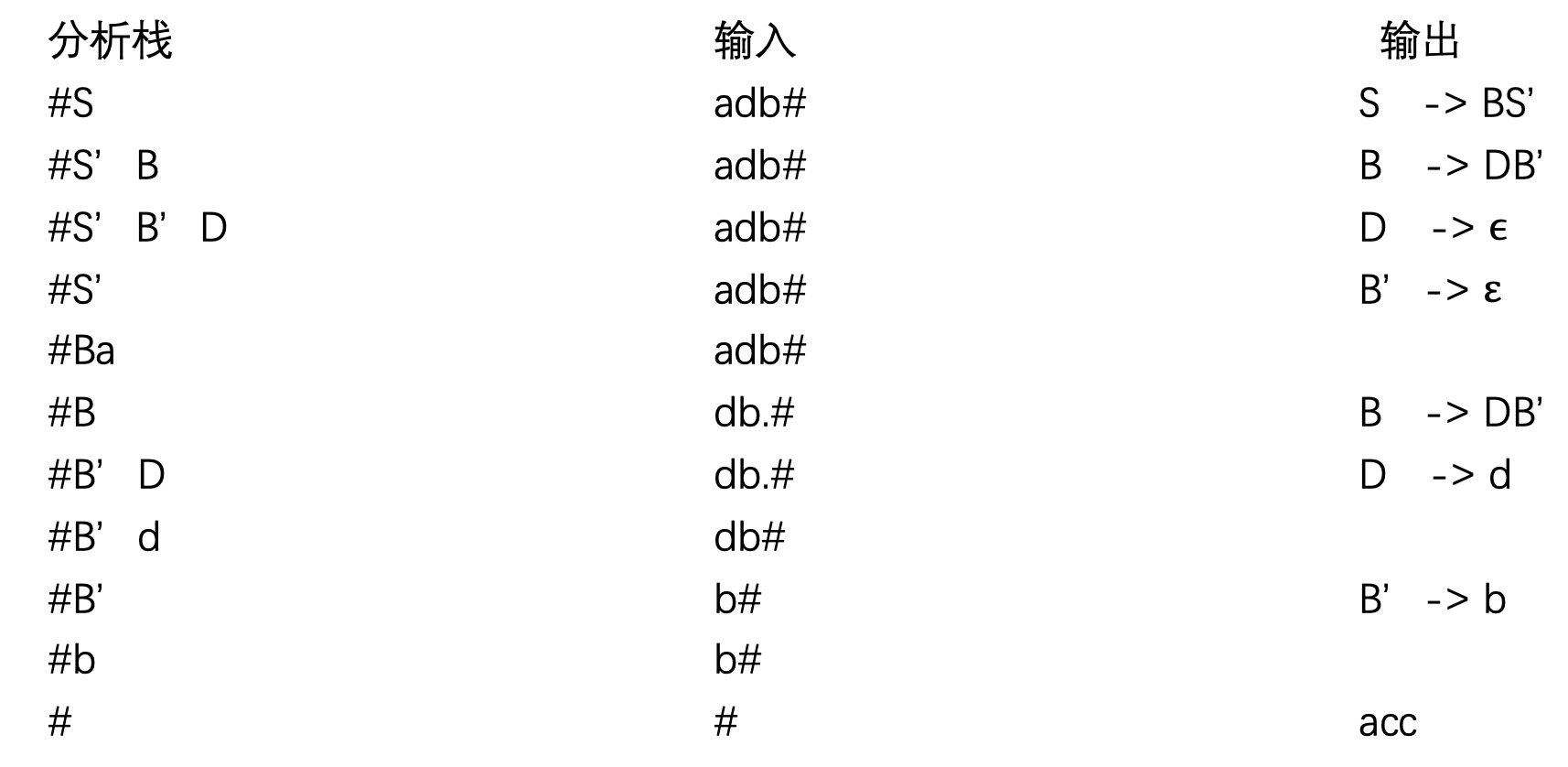解释： 将开始符号S放入栈顶，要分析的句子放入输入，看分析表，当S遇到a时，根据第三条规则，执行 S → \rightarrow BS ‘ ^ ,将S从栈顶逐出，将BS ‘ ^ 按反序推进栈，得到S ‘ ^ B，看分析条，当B遇到a，根据第三条规则，执行 B → \rightarrow DB ‘ ^ , 将B从栈顶逐出，将DB ‘ ^ 按反序推进栈，得到B ‘ ^ D, 看分析表 …(依此类推)，当分析栈为终结符时，则根据第二条规则，分析栈栈顶和输入串第一个字符进行抵消，当分析栈栈顶为‘#’，且输入串为‘#’时，则宣布分析成功，停止分析过程 展开全文FOLLOW集 • First集合的构造 计算文法符号X的First（X）时，不断应用下列规则，直到再没有新的终结符号或者ε可以被加入到First集合中为止： 1）如果X是一个终结符号，那么First(X)=X。 2）若X→…右边第一个符号是终结符或 ε ... First集合的构造 计算文法符号X的First（X）时，不断应用下列规则，直到再没有新的终结符号或者ε可以被加入到First集合中为止： 1）如果X是一个终结符号，那么First(X)=X。 2）若X→…右边第一个符号是终结符或 ε ，则直接将其加入 First（X）。 3）若X→…右边第一个符号是非终结符，则将其 First 集的的非 ε 元素加入 First（X）。 4）若X→…右边第一个符号是非终结符而且紧随其后的是很多个非终结符，这个时候就要注意是否有 ε 。 4.1]若第 i 个非终结符的 First 集有 ε ，则可将第 i+1 个非终结符去除 ε 的 First 集加入 First（X）。 4.2]若所有的非终结符都能够推导出 ε ，则将 ε 也加入到 First（X）。 现在我们可以按照如下方法计算任何串X1X2X3…Xn的First集合。向First（X1X2X3…Xn）加入First（X1）中所有的非ε符号。如果ε在First（X1）中，再加入First（X2）中所有的非ε符号；如果ε还在First（X2）中，再加入First（X3）中所有的非ε符号，以此类推。最后，如果ε在所有的First（Xi）中，那么将ε加入到First（X1X2X3…Xn）中。 Follow集合的构造 计算非终结符号A的First（A）时，不断应用下列规则，直到再没有新的终结符号可以被加入到任意Follow集合中为止： 1）将$放到Follow（S）中，其中S是开始符号，而 $是输入右端的结束标记。 2）如果存在一个产生式A→αBβ，那么First（β）中除了ε之外的所有符号都在Follow（B）中。 3）如果存在一个产生式A→αB，或存在产生式A→αBβ且First（β）包含ε，那么Follow（A）中所有符号都在Follow（B）中。 PS：α，β可以为空。 预测分析表的构造 消除左递归之后对于文法的每一个产生式A→α，进行如下处理： 1）对于First（α）中的每个终结符号a，将A→α加入到M【A,a】中。 2）如果ε在First（α）中，那么对于Follow（A）中的每一个终结符号b，将A→α加入到M【A,b】中。如果ε在First（α）中，且$在Follow（A）中，也将A→α加入到M【A， $】中。 在完成上面的操作后，如果M【A，a】中没有产生式，那么将M【A，a】设置为error（在表中用空条目表示）。 展开全文• FIRST集合 定义 可从α推导得到的串的首符号的集合，其中α是任意的文法符号串。 规则 计算文法符号 X 的 FIRST(X)，不断运用以下规则直到没有新终结符号或 ε可以被加入为止 ： （1）如果 X 是一个终结符号，... • 编译原理Java实现完整自顶向下语法分析——First、Follow、Select、判断LL(1)、提取公因子、消除左递归、自顶向下分析输入串 • FIRST集、FOLLOW集 和 SELECT集 一、FIRSTFIRST(A)为A的开始符或者首符号集。 1、定义： 设G=(VT，VN，S，P)是上下文无关文法 ，FIRST(α)={a|α能推导出aβ,a∈VT，α,β∈V*} 特别的，若α能...select集 follow集 • C和C++混合编写的编译原理实验，包括求first/follow/select,进行词法分析 构造预测分析表及判断是否为LL1文法 • 实验要求 设计一个程序，从任意字符串中剔除C语言...实验原理分析 按行读取，有两个字符变量，用来存储当前字符和上一个字符，若两个字符为“//”或者“/*”，则说明进入到注释部分，后面的数据都可以忽略，同时将空 • Note: suppose stmt-sequence.next=L0, that is, the label attached to the first three-address instruction to be executed after the code for stmt-sequence(the root of the syntax tree) will always be L0 ... • 里面是编译原理课上所讲的求first的集合的源代码，使用C++编写的 • First集：就是本非终结符的首部 Follow集：就是本非终结符的下一个终结符，如果后面没有就把左部非终结符的Follow集添加进来。 关于action表与goto表的计算，这个就是找闭包：点在中间就是移进项目，点在最后就是... • (1)可以求出任意给定文法的FIRST集和FOLLOW集（不含左递归和左公因子）（可在源代码主函数修改测试）。 (2)可以根据求出的FIRST和FOLLOW集求出预测分析表。 (3)可以根据预测分析表对某语句进行语法分析并输出分析... • 理解词法分析在编译程序中的作用；加深对有穷自动机模型的理解； 掌握词法分析程序的实现方法和技术。 实验内容 选择部分C语言的语法成分，设计其词法分析程序，要求能够识别关键字、运算符、分界符、标识符、常量... • 2、实现由 LL(1)文法构造 First 集和 Follow 集的算法。 3、根据First 集和 Follow 集构造相应的预测分析表。 4、实现预测分析技术的总控程序。 5、输出识别过程(推导或语法树)及结论。 【测试用例...Java 自上而下的语法分析 • 编译原理实验，包括词法分析，LL1文法，LR1文法三个实验，运行结果完整，词法分析结果展示清晰，LL1有预测分析表,first,fllow集，规约分析步骤界面显示，LR1y有动作表（ACTION）和状态转换（GOTO）以及项目集族构造... • 实验一：状态转换图 输入一串数据，利用状态转换图程序求出“关键字，标识符，整数，运算符，实数”。...实验三：first集，follow集计算 输入一个不含左递归的文法，由此程序求出该文法的first集和follow集。 • first集 定义 first(x)：可以从x推导出的所有串首终结符构成的集合 若x→ ε ，ε ∈first(x) follow集 定义 follow(A)是可能在某个句型紧跟在A后面终结符a的集合 如果A是某个句型的最右符号，则将终结符$加入到...
• 编译原理实验报告及源码，LL1 FIRST FOLLOW集 字符串匹配
• ## 编译原理实验代码

千次阅读 2019-04-04 20:36:55
编译原理实验代码（有Bug） /* #include "pch.h" #include "exe2.h" #include<memory> int main() { //终结符 list<string> terminator; terminator.push_back("a"); terminator.push_back("b"); ...
• 编译原理实验-LL1语法分析器（自动生成First、Follow）java 博主在做实验时，参考众多他人代码，发现bug众多，在[@moni_mm]代码基础上，与伙伴把能看到的BUG都做出修正，同时增添了一个GUI展示。再次我将代码做出...java 编译器...